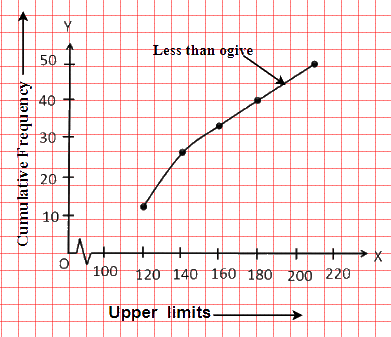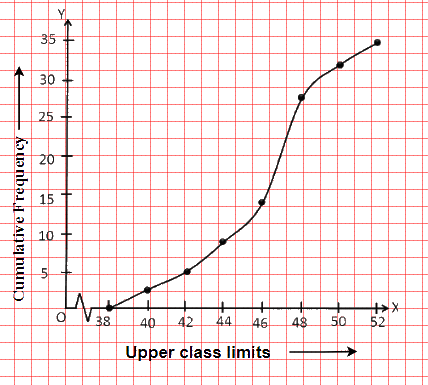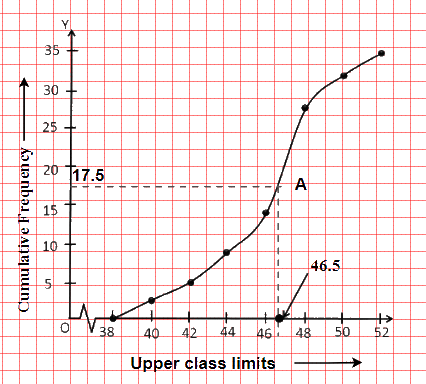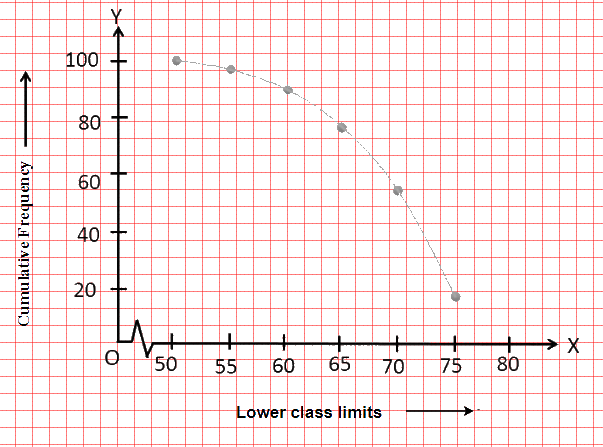# NCERT Solutions For Class 10 Maths Chapter 14 Exercise 14.4

Go back to  'Statistics'

## Chapter 14 Ex.14.4 Question 1

The following distribution gives the daily income of  $$50$$ workers of a factory.

 Daily income (in Rs) $$100 - 120$$ $$120 - 140$$ $$140 - 160$$ $$160 – 180$$ $$180 - 200$$ Number of workers $$12$$ $$14$$ $$8$$ $$6$$ $$10$$

Convert the distribution above to a less than type cumulative frequency distribution, and draw its ogive.

### Solution

What is known?

The daily income of $$50$$ workers of a factory.

What is unknown?

The less than type cumulative frequency distribution and its ogive.

Reasoning:

The representation of cumulative frequency distribution graphically is known as a cumulative frequency curve, or an ogive.

Steps:

The frequency distribution table of less than type is as follows:

 Daily income (in Rs) (Upper class Limits) Cumulative Frequency Less than $$120$$ $$12$$ Less than $$140$$ $$12 + 14 = 26$$ Less than $$160$$ $$26 + 8 = 34$$ Less than $$180$$ $$34 + 6 = 40$$ Less than $$200$$ $$40 + 10 = 50$$

Taking upper class limits of class intervals on $$x$$-axis and their respective frequencies on $$y$$-axis, its ogive can be drawn as follows:## Chapter 14 Ex.14.4 Question 2

During the medical check-up of $$35$$ students of a class, their weights were recorded as follows:

 Weight in (Kg) Number of students Less than $$38$$ $$0$$ Less than $$40$$ $$3$$ Less than $$42$$ $$5$$ Less than $$44$$ $$9$$ Less than $$46$$ $$14$$ Less than $$48$$ $$28$$ Less than $$50$$ $$32$$ Less than $$52$$ $$35$$

Draw a less than type ogive for the given data. Hence obtain the median weight from the graph and verify the result by using the formula.

### Solution

What is known?

The weight of $$35$$ students of a class.

What is unknown?

The less than type ogive and median weight.

Reasoning :

The representation of cumulative frequency distribution graphically is known as a cumulative frequency curve, or an ogive.

Steps:

The given cumulative frequency distributions of less than type are

 Weight in (Kg) Number of students Less than $$38$$ $$0$$ Less than $$40$$ $$3$$ Less than $$42$$ $$5$$ Less than $$44$$ $$9$$ Less than $$46$$ $$14$$ Less than $$48$$ $$28$$ Less than $$50$$ $$32$$ Less than 52 $$35$$

Taking upper class limits on $$x$$-axis and their respective cumulative frequencies on $$y$$-axis, its ogive can be drawn as follows.Here, $$n = 35$$  $$\Rightarrow \frac n{2}=17.5$$

Mark the point $$'A'$$ whose ordinate is $$17.5$$ and its $$x$$-coordinate is $$46.5.$$ Therefore, median of this data is $$46.5.$$It can be observed that the difference between two consecutive upper-class limits is $$2.$$ The class marks with their respective frequencies are obtained as below

 Weight (in Kg) Frequency Cumulative Frequency Less than $$38$$ 0 $$0$$ $$38$$ - $$40$$ $$3 - 0 = 3$$ $$3$$ $$40$$ - $$42$$ $$5 - 3 = 2$$ $$5$$ $$42$$ - $$44$$ $$9 - 5 = 4$$ $$9$$ $$44$$ - $$46$$ $$14 - 9 = 5$$ $$14$$ $$46$$ - $$48$$ $$28 - 14 = 14$$ $$28$$ $$48$$ - $$50$$ $$32 - 28 = 4$$ $$32$$ $$50$$ - $$52$$ $$35 - 32 = 3$$ $$35$$ Total $$n=35$$

Cumulative frequency $$(cf)$$ just greater than $$17.5$$ is $$28$$, beloging to class $$46-48.$$ Therefore,median class $$=46-48$$

Class size ($$h$$) $$= 2$$

Lower class limit of median class ($$l$$)$$=46$$

Frequency of median class ($$f$$)$$=14$$

Cumulative frequency of class preceding median class ($$cf$$) $$=14$$

\begin{align}\text{Median} &= l + \left( {\frac{{\frac{n}{2} - cf}}{f}} \right) \times h\\ &\!=\!46\!+\!\left( \!{\frac{{17.5 - 14}}{{14}}} \!\right)\!\!\times\!\!2\\ &= 46 + \frac{{3.5}}{7}\\ &= 46.5 \end{align}

Therefore, median of this data is $$46.5.$$

Hence, the value of median is verified.

## Chapter 14 Ex.14.4 Question 3

The following table gives production yield per hectare of wheat of $$100$$ farms of a village.

 Production yield(in kg/ha) $$50$$ – $$55$$ $$55$$– $$60$$ $$8$$ – $$65$$ $$65$$ – $$70$$ $$70$$ – $$75$$ $$75$$ – $$80$$ Number of farms $$2$$ $$8$$ $$12$$ $$24$$ $$38$$ $$16$$

Change the distribution to a more than type distribution and draw ogive.

### Solution

What is known?

Production yield per hectare of wheat of $$100$$ farms of a village.

What is unknown?

The more than type distribution and its ogive.

Reasoning:

The representation of cumulative frequency distribution graphically is known as a cumulative frequency curve or an ogive.

Steps:

The cumulative frequency distribution of more than type can be obtained as follows.

 Production Yield (in kg/ha) (Lower class limits) Cumulative Frequency More than or equal to $$50$$ $$100$$ More than or equal to $$55$$ $$100 - 2 = 98$$ More than or equal to $$60$$ $$98 - 8 = 90$$ More than or equal to $$65$$ $$90 - 12 = 78$$ More than or equal to $$70$$ $$78 - 24 = 54$$ More than or equal to $$75$$ $$54 - 38 = 16$$

Taking the lower-class limits on $$x$$-axis and their respective cumulative frequencies on $$y$$-axis, its ogive can be obtained as follows.